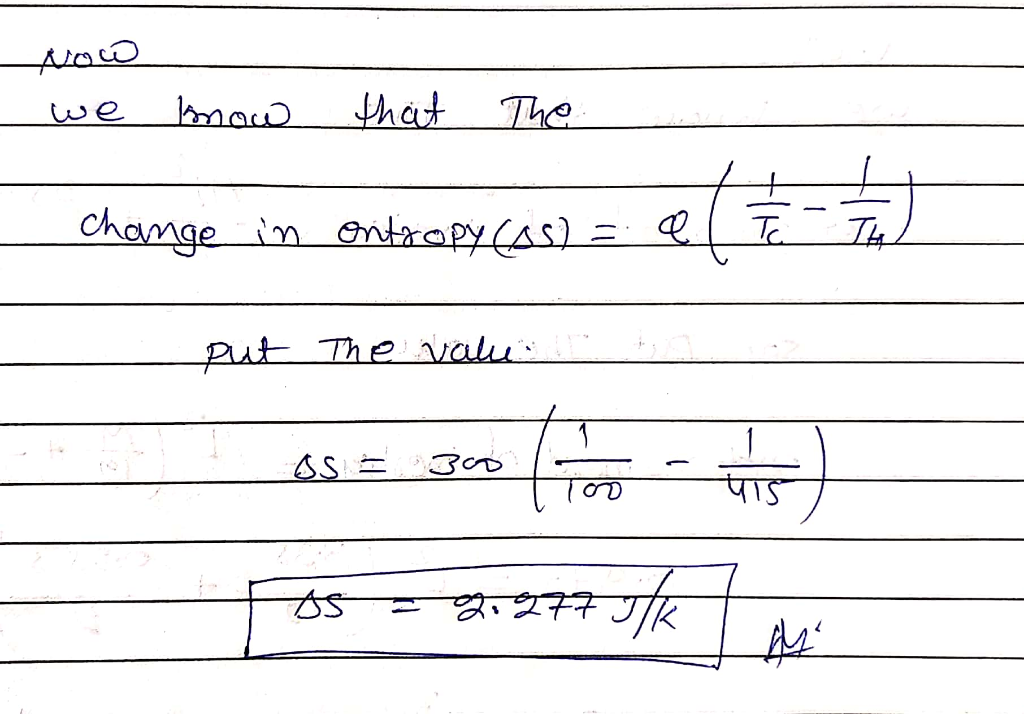In: Physics

# Suppose that 300.0 J of heat is transferred by conduction from a heat reservoir at a...

Suppose that 300.0 J of heat is transferred by conduction from a heat reservoir at a temperature of 415.0 K to another reservoir. Calculate the entropy change if the temperature of the second reservoir is 100.0 K

## Solutions

##### Expert Solution## Related Solutions

##### The reservoir temperatures for heat engines A through D are given below. Rank the engines in...
The reservoir temperatures for heat engines A through D are given below. Rank the engines in order of increasing efficiency. Indicate ties where appropriate. Engine A B C D TT hh (K)(K) 400 440 800 1240 TT cc (K)(K) 200 420 600 1020 Rank from largest to smallest. To rank items as equivalent, overlap them._
##### Write a function to solve the two-dimensional in Matlab, unsteady heat conduction equation with no internal...
Write a function to solve the two-dimensional in Matlab, unsteady heat conduction equation with no internal heat generation on a square domain. The length of each side of the square is 1 m. The function will have the following 4 inputs: npts                     number of grid points in each coordinate direction nt                         number of time steps to take dt                         size of time step (s) alpha                   thermal diffusivity (m2/s) Use the following initial and boundary conditions: Initialize the body to T =...
##### 1. Specific heat of water is 4.184 J/g ˚C. What is the heat in KJ gained...
1. Specific heat of water is 4.184 J/g ˚C. What is the heat in KJ gained when 20 q of water is heated from 22˚C to 77˚C? 2. What is the heat in J to melt 100g of NaCl when the HF for NaCl is 518 J/g? 3. What is the heat in J to equate 50 g Acetic Acid when the HE = 390 J/g?
##### A 62.5 g of iron with a heat capacity of .450 J/C is heated to 100...
A 62.5 g of iron with a heat capacity of .450 J/C is heated to 100 degree C. It is then transferred to a coffee cup calorimeter containing 52.7 g of water (specific heat of 4.184J/g C) initially at 20.63C. if the final temp of the system is 29.59 what was the heat capacity of the calorimeter?
##### A bacterial cell that transits from a reservoir or from one host to another, is likely...
A bacterial cell that transits from a reservoir or from one host to another, is likely to encounter a range of non-optimal conditions in its new environment. For example, it may be exposed to damaging or toxic physical and chemical conditions. It will need to adapt if it is to survive. Outline what these physical and chemical properties are and give examples of how bacteria adapt to survive in these conditions.
##### calculate the amount of J required to heat 115.0g of solid H2O at -25 degrees C...
calculate the amount of J required to heat 115.0g of solid H2O at -25 degrees C to liquid H20 at 30 degrees C
##### A sample of calcium carbonate [CaCO3 (s)] absorbs 45.5 J of heat, upon which the temperature...
A sample of calcium carbonate [CaCO3 (s)] absorbs 45.5 J of heat, upon which the temperature of the sample increases from 21.1C to 28.5C. If the specific heat of calcium carbonate is 0.82 J/g-K, what is the mass (in grams) of the sample? I have this answer: heat absorbed = mass of sample * specific heat of sample * change in temp of the sample 45.5 = mass * 0.82 * (28.5-21.1) mass = 7.5 g But my main question...
##### Air is supplied to a convergent–divergent nozzle from a reservoir where the pressure is 100 kPa....
Air is supplied to a convergent–divergent nozzle from a reservoir where the pressure is 100 kPa. The air is then discharged through a short pipe into another reservoir where the pressure can be varied. The cross-sectional area of the pipe is twice the area of the throat of the nozzle. Friction and heat transfer may be neglected throughout the flow. If the discharge pipe has constant cross-sectional area, determine the range of static pressure in the pipe for which a...
##### You are planning to buy a heat exchanger to recover 1.3 MMBtu/hr of waste heat from...
You are planning to buy a heat exchanger to recover 1.3 MMBtu/hr of waste heat from a process into a feed stream. Currently you pay $10/MMBtu for steam to heat up this feed stream. You can install a carbon steel shell and tube exchanger (U-tube type, with a heat transfer area of 150 m2) into a tight spot between two other pieces of equipment. This exchanger costs 199,757$ and will need to be replaced in 10 years, but will have...
##### A heat pump-bases HVAC system can remove heat from a building by (a) compressing the refrigerant...
A heat pump-bases HVAC system can remove heat from a building by (a) compressing the refrigerant to make it hotter than the outside air (b) compressing the refrigerant to make it cooler than the outside air (c) expanding the refrigerant to make it hotter than the outside air (d) blowing the heat out of the building using a fan